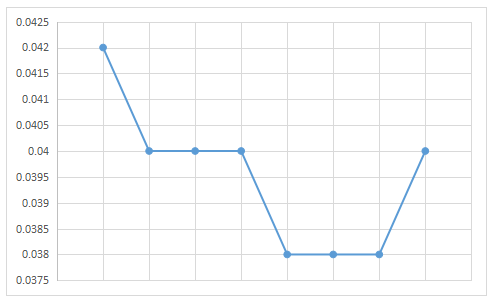### XAT 2011 Question 96

Instructions

Answer questions based on the following information:
Mulchand Textiles produces a single product of only one quality – waterproof synthetic fabric. Mr.Sharma, the cost accountant of Mulchand Textiles, estimated the costs of Mulchand Textiles for different possible monthly output levels. Before he could tabulate his estimates his computer crashed, and Mr. Sharma lost all data. Fortunately he had some printouts of some incomplete tables, charts and diagrams. The table titled “Variable Cost Estimates of Mulchand Textiles” provided the estimates of labour and material costs.

Apart from labour and material costs Mulchand Textiles incurs administrative costs of Rs. 40,000 per month, and electricity costs. Mr. Sharma recalled that estimate data of variable electricity cost had certain peculiar characteristics. Values at every 25000 sq ft of output increased in geometric progression till 150000 sq ft of output, after which values increased in arithmetic progression for every 25000 sq ft of output. Mr. Sharma remembered that the electricity cost was estimated to be Rs. 3800 for 25000 sq.ft. of output, Rs. 5700 for 50000 square feet of output and Rs. 38856.50 for 175000 square feet of output.

Question 96

# The estimated material cost given in the table titled “Variable Cost Estimates of Mulchand Textiles” included the cost of material that gets spoiled in the production process. Mr. Sharma decomposed the estimated material cost into material spoilage cost and material usage cost, but he lost the data when his computer crashed. When he saw the following line diagram, here called that he measured the estimate of material spoilage cost per square feet of output on the y - axis and monthly output on the x - axis.Estimated material usage cost per square feet of output.

Solution

Material cost for 25000 sq. ft output = Rs. 11050
Spoilage cost per sq. ft = 0.042 {Form the graph}

Therefore the spoilage cost for 25000 sq. ft = 25000*0.042 = Rs. 1050. Hence, the usage cost = 11050 - 1050 = Rs. 10000.

Therefore, the usage cost per sq. ft. = $$\dfrac{10000}{25000}$$ = Rs. 0.40.

Similarly, we can calculate for the remaining output and tabulating the same.

From the table we can see that that the usage cost per sq.ft is the same for all outputs. Hence, we can see that option C is the correct answer.